On this page, you will find Magnetic Effects of Electric Current Class 10 Notes Science Chapter 13 Pdf free download. CBSE NCERT Class 10 Science Notes Chapter 13 Magnetic Effects of Electric Current will seemingly help them to revise the important concepts in less time.

## CBSE Class 10 Science Chapter 13 Notes Magnetic Effects of Electric Current

### Magnetic Effects of Electric Current Class 10 Notes Understanding the Lesson

When an electric current is passed through a conductor, a magnetic field is produced around it.

1. Magnetic field

• The region surrounding a magnet, in which the force of the magnet can be detected, is said to have a magnetic field.
• Magnetic field is a quantity that has both direction and magnitude.
• The direction of the magnetic field is taken to be the direction in which a north pole of the compass needle moves inside it.

2. Magnetic field lines

• Magnetic field lines- are the imaginary lines along which the iron filings align themselves.
• By convention, the field lines emerge from the north pole and merge at the south pole. Inside the magnet, the direction of field lines is from the south pole to the north pole.

Properties of Magnetic field line

• Magnetic field lines are closed curves.
• The relative strength of the magnetic field is shown by the degree of closeness of the field lines.
• No two field lines can cross each other as at the point of intersection the compass needle would point towards two directions, which is not possible.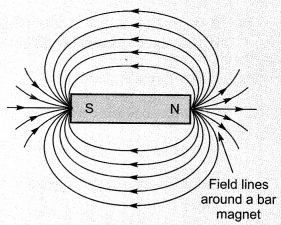3. Magnetic field due to current carrying conductor

• The magnetic field around a current carrying conductors forms a pattern of concentric circles.
• The magnitude of the magnetic field produced at a given point increases as the current through the wire increases.
• The magnetic field produced by a given current decreases as the distance from it increases.

4. Right-hand thumb rule: The direction of magnetic field produced by a current carrying conductor can be found out by using the right-hand thumb rule.

Imagine that you are holding a current-carrying straight conductor in your right hand such that the thumb points towards the direction of current. Then your fingers will wrap around the conductor in the direction of the field lines of the magnetic field, as shown in Figure. This is known as the right hand thumb rule.(a) A pattern of concentric circles indicating the field lines of a magnetic field around a straight conducting wire. The arrows in the circles show the direction of the field lines. (b) A close up of the pattern obtained.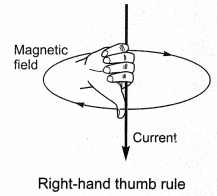5. Magnetic field due to current-carrying circular loop

• At the centre of a current-carrying loop, the magnetic field appears to be a straight in line.
• The magnetic field produced by a current-carrying wire at a given point depends directly on the current passing through it.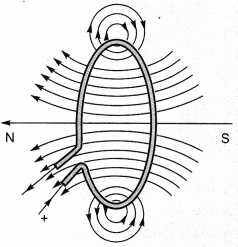6. Magnetic field due to a solenoid
Solenoid: A coil of many circular turns of insulated copper wire wrapped closely in the shape of a cylinder is called a solenoid.

• The pattern of the magnetic field around a current-carrying solenoid is same as that of the bar magnet. One end of the solenoid behaves as a magnetic north pole, while the other behaves as south pole.
• The field lines inside the solenoid are in the form of parallel straight lines.
• The field is uniform inside the solenoid.
• A strong magnetic field produced inside a solenoid can be used to magnetise a piece of magnetic material, like soft iron, when placed inside the coil. The magnet so formed is called an electromagnet.7. Force on a current-carrying conductor in a magnetic field

• A current carrying conductor when placed in a magnetic field experiences a force.
• If the direction of the field and that of the current are mutually perpendicular to each other, then the force acting on the conductor will be perpendicular to both and will be given by Fleming’s left hand rule.
• Fleming’s left-hand rule: According to this rule, stretch the thumb, forefinger and middle finger of your left hand such that they are mutually perpendicular. If the forefinger points in the direction of magnetic field and the middle finger in the direction of current, then the thumb will point in the direction of the force acting on the conductor.8. Electric motor: An electric motor is a rotating device that converts electrical energy to mechanical energy.
Principle: A current carrying conductor when placed in a magnetic field experiences a force.
Commercial motors are made up of:

• an electromagnet in the place of permanent magnet.
• large number of turns of the conducting wire in the current carrying coil.
• a soft iron core on which the coil is wound.

Electromagnetic induction: The phenomenon of electromagnetic induction is the production of induced current in a coil placed in a region where the magnetic field changes with time.

• The magnetic field may change due to relative motion between the coil and the magnet.• If the coil is placed near a current carrying conductor, the magnetic field may change either due to a change in the current through the conductor or due to the relative motion between the coil and conductor.
•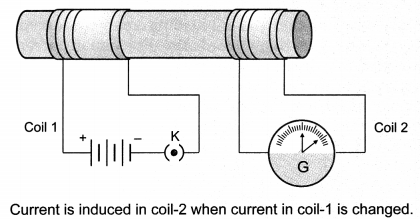Fleming’s right-hand rule to know the direction of the induced current. Stretch the thumb, forefinger and middle finger of the right hand so that they are perpendicular to each other, as shown in the figure. If the forefinger indicates the direction of the magnetic field and the thumb shows the direction of motion of the conductor, then the middle finger will show the direction of induced current. This simple rule is called Fleming’s right-hand rule.Fleming’s right-hand rule.
A galvanometer is an instrument that can detect the presence of a current in a circuit. The pointer remains at zero (the centre of the scale) for zero current flowing through it. It can deflect either to the left or to the right of the zero mark depending on the direction of current.
The induced current is found to be the highest when the direction of motion of the coil is at right angles to the magnetic field.

Electric Generator: A generator converts mechanical energy into electrical energy.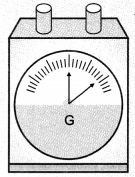Principle: It works on the principle of electromagnetic induction.
AC generator produces AC current and DC generator produces DC current.

9. AC and DC

• The difference between the direct and alternating currents is that the direct current always flows in one direction, whereas the alternating current reverses its direction periodically.
• In India, the AC changes direction after every 1/100 second, that is, the frequency of AC is 50 Hz.
• An important advantage of AC over DC is that electric power can be transmitted over long distances without much loss of energy.

10. Domestic Electric circuits

• In our houses we receive AC electric power of 220 V with a frequency of 50 Hz. One of the wires in this supply is with red insulation, called live wire. The other one is of black insulation, which is a neutral wire. The potential difference between the two is 220 V. The third is the earth wire that has green insulation and this is connected to a metallic body deep inside the earth. It is used as a safety measure to ensure that any leakage of current to a metallic body does not give any severe shock to a user.
• Fuse is the most important safety device, used for protecting the circuits due to short-circuiting or overloading of the circuits.

### Class 10 Science Chapter 13 Notes Important Terms

Magnetic field: The area around a magnet in which its magnetic force can be experienced.

Right-Hand Thumb Rule: Imagine that you are holding a current carrying straight conductor in your right hand such that the thumb is pointing towards the direction of current. Then the fingers wrapped around the conductor will give the direction of the magnetic field.

Solenoid: A coil of many circular turns of insulated copper wire wrapped closely in a cylindrical form.

Electromagnet: An electomagnet is a type of magnet in which the magnetic field is produced by an electric current. The magnetic field disappears when the current is turned off.

Felming’s Left-Hand Rule: Stretch the thumb, forefinger and middle finger of your left hand such that they are mutually perpendicular. If the forefinger points in the direction of magnetic field, the middle finger in the direction of current then the thumb will point in the direction of motion or force.

Electromagnetic Induction: When a conductor is placed in a changing magnetic field, some current is induced in it. Such current is called induced current and the phenomenon is called electromagnetic induction.

Fleming’s Right-Hand Rule: Hold the thumb, the forefinger and the middle finger of right hand at right angles to each other. If the forefinger is in the direction of magnetic field and the thumb points in the direction of motion of the conductor, then the direction of induced current is indicated by the middle finger.

Electric motor: An electric motor is a rotating device that converts electrical energy to mechanical energy.

Electric generator: A generator converts mechanical energy into electrical energy.

Alternate Current (A.C.): The current which reverses its direction periodically.

Direct Current (D.C.): The current which flows in one direction only.

Earth Wire: Protects us from electric shock in case of leakage of current especially in metallic body appliances. It provides a low resistance path for current in case of leakage of current.

Short Circuit: When live wire comes in direct with neutral wire accidently.